Maths-
General
Easy

Question

# Factor the polynomial as the product of binomials.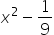Hint:

## The correct answer is: (x-1/3)(x+1/3) is the required product of binomials.

### Ans :-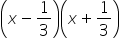is the required product of binomials.Given ,Write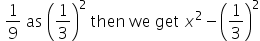As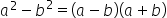Here a = x and b =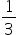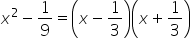∴is the required product of binomials.#### With Turito Foundation.#### Get an Expert Advice From Turito.